# Multifractals

## 11/28/00 Lecture #13 in Physics 505

###Comments on Homework #11 (Nonlinear Prediction)

• Results varied from almost too good to rather poor
• Hard to diagnose the poor results when not enough detail given
• You could try several data sets to see how general are your results
• Here's sample results (averaged over 1000 realizations of the Hénon map):

•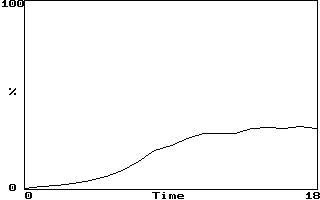###Review (last week) - Calculation of Fractal Dimension

• Cover dimension
• Find the number N of (hyper)cubes of size d required to cover the object
• D0 = -d log(N) / d log(d)
• Capacity dimension
• Similar to cover dimension but cubes are fixed in space
• D0 = -d log(N) / d log(d)
• This derivative should be taken in the limit d --> 0
• Many data points are required to get a good result

• The number required increases exponentially with D0
• Examples of fractals
• Some ways to produce fractals by computer:
• Chaotic dynamical orbits (strange attractors)
• Recursive programming
• Iterated function systems (the chaos game)
• Infinite mathematical sums (Weierstrass function, etc.)
• Boundaries of basins of attraction
• Escape-time plots (Mandelbrot, Julia sets, etc.)
• Random walks (diffusion)
• Diffusion limited aggregation
• Cellular automata (game of life, etc.)
• Percolation (fluid seeping through porous medium)
• Self-organized criticality (avalanches in sand piles)
• Similarity dimension
• Example #1  (Sierpinski carpet):

•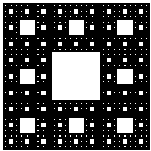D = log(8) / log(3) = 1.892789261...
• Example #2  (Koch curve):

•D = log(4) / log(3) = 1.261859507...
• Example #3  (Triadic Cantor set):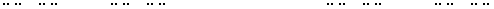• D = log(2) / log(3) = 0.630929753

###Correlation Dimension

• Capacity dimension does not give accurate results for experimental data
• Similarity dimension is hard to apply to experimental data
• The best method is the (two-point) correlation dimension (D2)
• This method opened the floodgates for identifying chaos in experiments
• Homework this week asked you to calculate D2 for the Hénon map
• Original (Grassberger and Procaccia) paper included with HW #12
• Illustration for 1-D and 2-D data embedded in 2-D
• Procedure for calculating the correlation dimension:
• Choose an appropriate embedding dimension DE
• Choose a small value of r (say 0.001 x size of attractor)
• Count the pairs of points C(r) with Dr < r
• Dr = [(Xi - Xj)2 + (Xi-1 - Xj-1)2 + ...]1/2
• Note: this requires a double sum (i, j) ==> 106 calculations for 1000 data points
• Actually, this double counts; can sum j from i+1 to N
• In any case, don't include the point with i = j
• Increase r by some factor (say 2)
• Repeat count of pairs with Dr < r
• Graph log C(r) versus log r (can use any base)
• Fit curve to a straight line
• Slope of that line is D2
• Think of C(r) as the probability that 2 random points on the attractor are separated by < r
• Correlation dimension emphasizes regions of space the orbit visits
• ExampleC(r) versus r for Hénon map with N = 1000 and DE = 2
• Result:  D2 = 1.223 ± 0.097
• Compare:  DGP = 1.21 ± 0.01 (Original paper, N = 15,000)
• See also my calculations (D2 = 1.220 ± 0.036) with N = 3 x 106
• Compare:  DKY = 1.2583  (from Lyapunov exponents)
• Compare:  D0 = 1.26  (published result for capacity dimension)
• Generally  D2 < DKY<D0  (but they are often close)

###Practical Considerations

• Tips for speeding up the calculation (roughly in order of increasing difficulty and decreasing value):
• Avoid double counting by summing j from i+1 to N
• Collect all the r values at once by binning the values of Dr
• Avoid taking square roots by binning Dr2 and using log x2 = 2 log x
• Avoid calculating log by using exponent of floating point variable
• Collect data for all embeddings at once
• Sort the data first so you can quit testing when Dr exceeds r
• Can also use other norms, but accuracy suffers
• Discard a few temporally adjacent points if sample time is small for flows
• Number of data points needed to get valid correlation dimension
• Need a range of r values over which slope is constant (scaling region)
• Limited at large r by the size of the attractor (D2 = 0 for r > attractor size)
• Limited at small r by statistics (need many points in each bin)
• Various criteria, all predict N increases exponentially with D2
• Tsonis criterion:  N ~ 10 2 + 0.4D  (D to use is probably D2)
•    D N 1 250 2 630 3 1600 4 4000 5 10,000 6 25,000 7 63,000 8 158,000 9 400,000 10 1,000,000
• Effect of round-off errors
• Round-off errors discretize the state space
• Causes the measured dimension to approach zero at small r
• This limits the useful scaling region of log C(r) versus log r
• Easy to test for this in low D since points will be identical
• Effect of superimposed noise
• Distinguishing chaos from noise
• Chaos has dimension less than infinity
• It may be unmeasurably high, however
• White noise has infinite dimension
• Colored (correlated) noise can appear low dimensional
• Example:  1/f 2 (brown) noise
•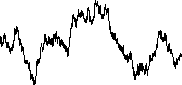• gives C(r) versus r with no real scaling region
• Dimension is low at large r, high at small r
• Osborne & Provenzale (Physica D 35, 357, 1989) show 1/f a noise tends to give D2 ~ 2 / (a - 1) for 1 < a < 3
• Conjecture:  It's possible to get any C(r) with appropriately colored noise
• Example: Triadic Cantor set (D = 0.631)

•• Visit the points on the attractor in random order
• Geometry is accurately fractal, dynamics is random
• Hence dimension can be low and well defined, but no chaos
• This would presumably fail at sufficiently high embedding
• A good demonstration of this would be a publishable student project
• K-S entropy (Kolmogorov-Sinai)
• Entropy is the sum of the positive Lyapunov exponents (Pesin Identity)
• A periodic orbit (zero LE) has 0 entropy (no spreading)
• A random orbit (infinite LE) has infinity entropy (maximal disorder)
• This is related to but different from the thermodynamic entropy
• It is actually a rate of change of the usual entropy
• Can be estimated from C(r, DE) in different embeddings
• Formula:  K = d log C(r)/dDE in the limit of infinite DE
• Multivariate data
• Suppose you have measured 2 (or more) simultaneous dynamical variables (X and Y)
• If they are independent, they reduce the required number of time delays
• Construct embedding from Xi, Yi, Xi-1, Yi-1, etc...
• Trick:  Make single time series by intercalation
• Xi, Yi, Xi+1, Yi+1, ... and use existing D2 algorithm
• Probably good to rescale variables to the same range
• Get twice as much data this way in the same time
• I'm not aware of this being discussed in literature but it works
• This could also be a publishable student project
• Filtered data
• What happens if you measure dX/dt or integral of X?
• This has been examined theoretically and numerically
• Differentiating is usually OK but enhances noise and lowers correlation time
• Integrating suppresses noise and increases correlation time - hard to get a good plateau in C(r)
• These operations can raise the dimension by 1 (adds an equation)
• Other filtering methods have not been extensively studied (another possible student project)
• Missing data
• What if some data points are missing or clearly in error?
• Honest method:
• Restart the time series
• You lose DE - 1 points on each restart
• Don't calculate across the gap
• But you can combine the segments into one C(r)
• Other options (if gap is of short duration):
• Ignore the gap (probably a bad idea)
• Set data to zero or to average (also a bad idea)
• Interpolate or curve fit (OK if data is from a flow)
• Use a nonlinear predictor to estimate the missing points (best idea)
• None of these work if gap is of long duration
• Nonuniformly sampled data
• If sampling is deterministically nonuniform:
• All is probably OK
• Dimension may increase since additional equations come into play
• If sampling is random:
• This will give infinite dimension if randomness is white
• If data is from a flow, and sample intervals are known, you can try to construct a data set with uniform intervals by interpolation or curve fitting
• How accurate does sample time have to be?  (good student project)
• Lack of stationarity
• dx/dt = F(x, y)
• dy/dt = G(x, y, t)
• dz/dt = 1  (non-autonomous slowly growing term)
• Increases system dimension by 1
• Increases attractor dimension by < 1
• If t is periodic, attractor projects onto a torus
• Can try to detrend the data
• This is problematic
• How best to detrend? (polynomial fit, sine wave, etc.)
• What is interesting dynamics and what is uninteresting trend?
• Take log first differences: Yn = log(Xn) - log(Xn-1) = log(Xn/Xn-1)
• Surrogate data
• Generate data with same power spectrum but no determinism
• This is colored noise
• Take Fourier transform, randomize phases, inverse Fourier transform
• Compare C(r), predictability, etc.
• Many surrogate data sets allow you to specify confidence level

###Multifractals

• Most attractors are not uniformly dense
• Orbit visits some portions more often than others
• Local fractal dimension may vary over the attractor
• Capacity dimension (D0) weights all portions equally
• Correlation dimension (D2) emphasizes dense regions
• q = 0 and 2 are only two possible weightings
• Let Cq(r) = S [ S q(r - Dr) / (N - 1)]q-1 / N
• Then Dq = [d log Cq(r)/d log r] / (q - 1)
• Note: for q = 2 this is just the correlation dimension
• q = 0 is the capacity dimension
• q = 1 is the information dimension
• Other values of q don't have names (so far as I know)
• q = infinity is dimension of densest part of attractor
• q = -infinity is dimension of sparsest part of attractor
• In general Dq < Dq-1

•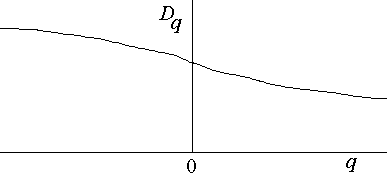• The K-S entropy can also be generalized

• Kq = -log S piq / (q - 1)N

###Summary of Time-Series Analysis

• Verify integrity of data
• Graph X(t)
• Correct bad or missing data
• Establish stationarity
• Observe trends in X(t)
• Compare first and second half of data set
• Detrend the data (if necessary)
• Take first differences
• Fit to low-order polynomial
• Fit to superposition of sine waves
• Examine data plots
• Xi versus Xi-1
• Phase space plots (dX/dt versus X)
• Return maps (max X versus previous max X, etc.)
• Poincaré sections
• Determine correlation time or minimum of mutual information
• Look for periodicities (if correlation time decays slowly)
• Use FFT to get power spectrum
• Use Maximum entropy method (MEM) to get dominant frequencies
• Find optimal embedding
• False nearest neighbors
• Saturation in correlation dimension
• Determine correlation dimension
• Make sure log C(r) versus log r has scaling (linear) region
• Make sure result is insensitive to embedding
• Make sure you have sufficient data points (Tsonis)
• Determine largest Lyapunov exponent and entropy (if chaotic)
• Determine growth of unpredictability
• Try to remove noise (if dimension is too high)
• Integrate data
• Use nonlinear predictor
• Use principal component analysis (PCA)
• Construct model equations
• Make short-term predictions
• Compare with surrogate data sets

###Time-Series Analysis Tutorial (using CDA)

• Sine wave
• Two incommensurate sine waves
• Logistic map
• Hénon map
• Lorenz attractor
• White noise
• Mean daily temperatures
• Standard & Poor's Index of 500 common stocks